# Moving Average (MA) Explained for Traders

## Introduction to Moving Averages: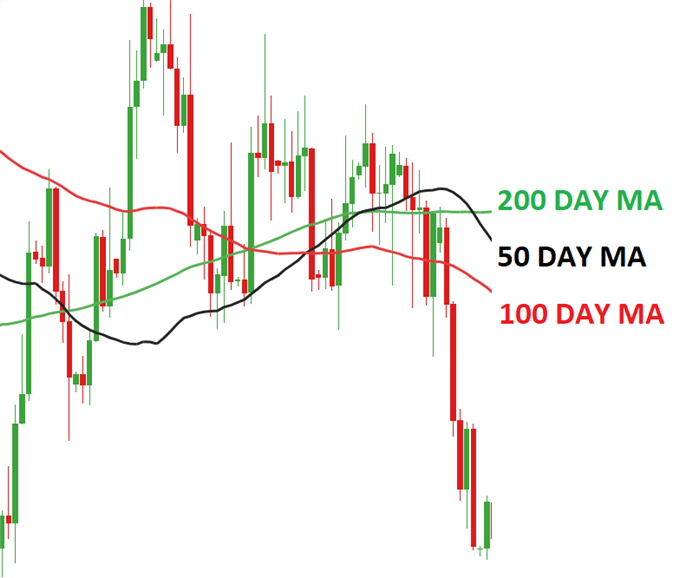Moving Average – Talking Points:

• What is a moving average?
• How do you calculate moving average?
• What is the purpose of moving averages?
• How do you interpret moving averages?

## What is a Moving Average?

In technical analysis, the moving average is an indicator used to represent the average closing price of the market over a specified period of time. Traders often make use of moving averages as it can be a good indication of current market momentum.

The two most commonly used moving averages are the simple moving average (SMA) and the exponential moving average (EMA). The difference between these moving averages is that the simple moving average does not give any weighting to the averages in the data set whereas the exponential moving average will give more weighting to current prices.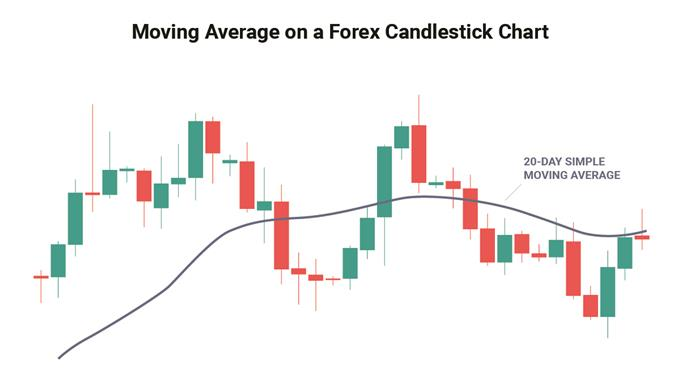## How do you calculate moving average?

As explained above, the most common moving averages are the simple moving average (SMA) and the exponential moving average (EMA). Almost all charting packages will have a moving average as a technical indicator.

The simple moving average is simply the average of all the data points in the series divided by the number of points.

The challenge of the SMA is that all the data points will have equal weighting which may distort the true reflection of the current market’s trend.

The EMA was developed to correct this problem as it will give more weighting to the most recent prices. This makes the EMA more sensitive to the current trends in the market and is useful when determining trend direction.

The mathematic formula for each can be found below:

Simple Moving Average:

SMA =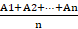Where:

A= Is each of the data points

n = Number of time periods

For example, looking at a 5-day SMA on a daily chart of EUR/USD and the closing prices over the 5 days are as follows:

Day 1: 1.321

Day 2: 1.301

Day 3: 1.325

Day 4: 1.327

Day 5: 1.326

SMA = (1.321 + 1.301 + 1.325 + 1.327 + 1.326)/5

SMA = 6.6/5

SMA = 1.32

Exponential Moving Average:

EMA =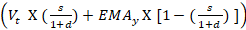Where:

EMAt= EMA today

Vt= Value today

EMAt= EMA today

s =smoothing

d = number of days

Steps for calculating EMA:

1. Calculate the SMA for the particular time period

2. Calculate the multiplier for weighting the EMA using the formula:

[2 ÷ (selected time period + 1)]. So, for a 10-day moving average, the multiplier would be [2/(10+1)]= 0.01818.

3. Use the smoothing factor combined with the previous EMA to arrive at the current value.

For example, looking at a 10-day EMA for a share, the table below displays how the EMA would be calculated:

 Date Price 10-day SMA Smoothing Constant 2/(10 + 1) 10-day EMA 1 24-Apr-18 23.24 2 25-Apr-18 22.99 3 26-Apr-18 22.85 4 27-Apr-18 23.00 5 28-Apr-18 22.96 6 29-Apr-18 22.21 7 30-Apr-18 21.99 8 1-May-18 22.43 9 2-May-18 22.24 10 3-May-18 22.55 22.65 22.65 11 4-May-18 22.15 22.54 0.1818 22.56 12 5-May-18 22.39 22.48 0.1818 22.53 13 6-May-18 22.38 22.43 0.1818 22.50 14 7-May-18 22.61 22.39 0.1818 22.52 15 8-May-18 23.36 22.43 0.1818 22.67 16 9-May-18 24.05 22.62 0.1818 22.92 17 10-May-18 23.75 22.79 0.1818 23.07 18 11-May-18 23.83 22.93 0.1818 23.21 19 12-May-18 23.95 23.10 0.1818 23.35 20 13-May-18 23.63 23.21 0.1818 23.40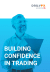Recommended by Tammy Da Costa

## What is the purpose of moving averages?

The main purpose of the moving average is to eliminate short-term fluctuations in the market. Because moving averages represent an average closing price over a selected period of time, the moving average allows traders to identify the overall trend of the market in a simple way.

Another benefit of the moving average is that it is a customizable indicator which means that the trader can select the time-frame that suits their trading objectives. Moving Averages are often used for market entries as well as determining possible support and resistancelevels. The moving average often acts as a resistance level when the price is trading below the MA and it acts as a support level when the price is trading above the MA.

## How do you interpret moving averages?

There are 3 ways in which trader’s use the moving average:

• To determine the direction of the trend
• To determine support and resistance levels
• Using multiple moving averages for long- and short-term market trends

1. To determine the direction of the trend:

When prices are trending higher, the moving average will adjust by also moving higher to reflect the increasing prices. This could be interpreted as a bullish signal, where traders may prefer buying opportunities.

The opposite would be true if the price was consistently trading below the moving average indicator, where traders would then prefer selling opportunities due to the market signaling a downward trend.

2. The moving average for support and resistance levels:

The moving average can be used to determine support and resistance levels once a trader has placed a trade.

If the trader sees the moving average trending higher, they may enter the market on a retest of the moving average. Likewise, if the trader is already long in an uptrend market, then the moving average can be used as a stop loss level. The opposite is true for down trends.

The charts below are examples of how the moving average can be used as a both a support and a resistance level.

3.Making use of multiple moving averages

It is common for traders to make use of multiple moving average indicators on a single chart, as depicted in the chart below. This allows traders to simultaneously assess the short and long-term trends in the market. As price crosses above or below these plotted levels on the graph it can be interpreted as either strength or weakness for a specific currency pair.This method of using more than one indicator can be extremely useful in trending markets and is similar to using the MACD oscillator.

When making use of multiple moving averages, many traders will look to see when the lines will cross. This phenomenon is referred to as ‘The Golden Cross’ when a bullish pattern is formed and ‘The Death Cross’ when the pattern is bearish.

A Golden cross is identified when the short-term moving average (such as the 50-day moving average) crosses above the long-term moving average (such as the 200-day moving average), while the Death cross represents the short-term moving average crossing below the long-term moving average. Traders that are long, should view a Death Cross as a time to consider closing the trade while those in short trades should view the Golden Cross as a signal to close out the trade.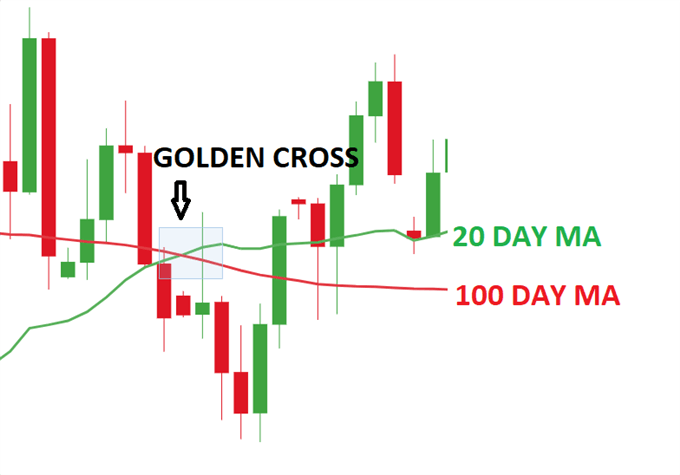## Moving Average Indicator: A Summary

In summary, the Moving Average is a common indicator used by traders to determine trends in the market. Many traders use more than one Moving Average at a time as this gives a more holistic view of the market. Moving averages are often used to determine market entries as well as support and resistance levels.